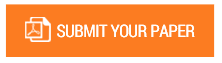Closed Form Stability Analysis of Solid Non-Prismatic Columns

Authors : Ibearugbulem O. M., Ezeh J. C., Ibarugbulem C. N., Onyechere I. C.

Volume/Issue : Volume 5 - 2020, Issue 3 - March

Scribd : https://bit.ly/39SN6g6

This paper presents the closed form stability analysis of solid non-prismatic columns. Using fundamental kinematics and Hooke’s law, the total potential energy functional of a non-prismatic column was obtained. This was minimized with respect to deflection and the non-linear Euler-Bernoulli equation of equilibrium of a non-prismatic column was obtained. Two mathematical axioms were employed to completely integrate the non-linear governing equation. Individual deflection equations for four columns of various boundary conditions were obtained. Substituting the deflection equation into the non-linear governing equation and rearranging it gave the closed form formula for calculating buckling loads of non-prismatic columns. This formula was used to determine buckling loads for eight example problems. Results from four of the example problems were compared with results from earlier study that used an approximate method called weighted moment of inertia. The highest percentage difference recorded is 9.59%, which validates the present method since the result from earlier study is based on approximate method.

Keywords : Closed form; non-prismatic; deflection; governing equations; buckling.

#### CALL FOR PAPERS

Paper Submission Last Date
31 - December - 2023